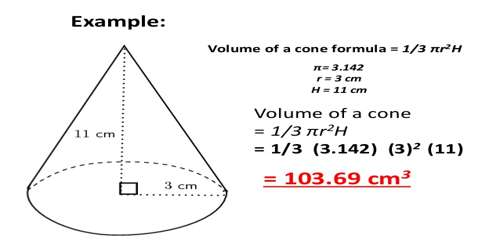Volume of Cone

A cone is a three-dimensional figure with one circular base. It has a curved surface which tapers (i.e. decreases in size) to a vertex at the top. The height of the cone is the perpendicular distance from the base to the vertex. A curved surface connects the base and the vertex. The volume of a 3 -dimensional solid is the amount of space it occupies.  Volume is measured in cubic units ( in3, ft3, cm3, m3,in3, ft3,cm3, m3, etc). Be sure that all of the measurements are in the same unit before computing the volume.

The volume of a right cone is equal to one-third the product of the area of the base and the height. It is given by the formula:

The volume of a cone is 1/3(Area of Base)(height) = 1/3 π r2

where r is the radius of the base and h is the perpendicular height of the cone.Example:

Calculate the volume if r = 2 cm and h = 3 cm

VCone = 1/3 × 3.14 × 22 × 3

VCone = 1/3 × 3.14 × 4 × 3

VCone = 1/3 × 3.14 × 12

VCone = 1/3 × 37.68

VCone = 1/3 × 37.68/1

VCone = (1 × 37.68)/(3 × 1)

VCone = 37.68/3 = 12.56 cm3

The volumes of a cone and a cylinder are related in the same way as the volumes of a pyramid and a prism are related. If the heights of a cone and a cylinder are equal, then the volume of the cylinder is three times as much as the volume of a cone.

Information Source: